HomeShow Me The Physics Lesson

Have a little Physics with your Pancakes

ReviewFill in the Blank Notes

I.) Electric Potential - work needed or energy acquired when a positive charge is moved from infinity to a certain point in an electric field.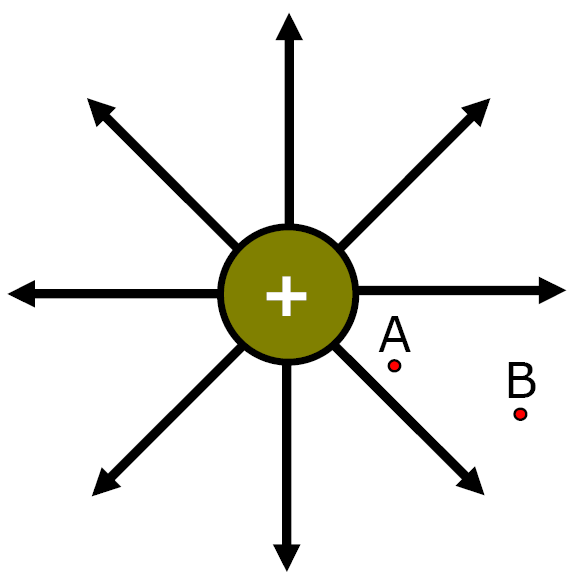II) Potential Difference (Voltage) potential energy difference between 2 points in an electric field per unit of chargeA) Potential Difference, Energy & Work

As a charge moves through an electric field it gains PE or KEIf "Opposites" move

CloserGain KE or PE?

They Gain KE

If 'Opposites' Separate

Gain KE or PE?

They Gain PE

If "Likes" SeparateGain KE or PE?

They Gain KE

If 'Likes' move closer

Gain KE or PE?

They Gain PE

1) Equation #1

Charge q moving between 2 points in a field

 V = W (Joules) q (Coulombs)

W = work done against field or energy acquired working with field (Joules or eV)

eV = electronVolts

(small energy unit)

q - amount of charge moving through field (Coulombs)

V - Potential Difference (volts)

AP Physics

Charge q moving between 2 points in a field

UE = PE (W)
 V = UE (Joules) q (Coulombs)

UE = qV =
PE between between 2 charges
 UE = kq1q2 r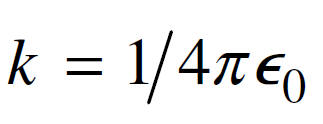V = Kq r
V - electric potential
(Scalar)

 Ex) It takes 6 J of work to move 2 C of charge between 2 points in an electric field.   What is the potential energy difference (V) between these points?

Ex) It takes 6 Joules of work to move 2 coulombs of charge between 2 points in an electric field. What is the potential energy difference (voltage) between these 2 points?

 V = W (Joules) q (Coulombs)

 V = 6 Joules 2 Coulombs

V = 3 Volts or J/C

2) Potential difference Eq. #2

 V = W (eV) q (elementary charges)

W = work done against field or energy acquired working with field

(Electronvolts = Ev)

Electronvolt
� small energy unit = 1.6 x 10-19 J

q - amount of charge moving through field (# elementary charges)

V - Potential Difference (volts)

 Ex) It takes 10 eV to move 2 elementary charges from one point to another in an electric field. Find the potential difference between these points.

Ex) It takes 10 eV to move 2 elementary charges from one point to another in an electric field. Find the potential difference between these points.

 V = W (eV) q (elementary charges)

 V = 10 eV 2 elementary charges

V = 5 Volts

 Ex) +2 elementary charges acquire 16 joules of energy when they move between 2 points in an electric field. What is the potential difference (voltage) between these two points in the field?

Ex) +2 elementary charges acquire 16 joules of energy when they move between 2 points in an electric field. What is the potential difference (voltage) between these two points in the field?

Units don't match!!!

elementary charges, eV   Joules/Coulombs

+2 elementary charges =

3.2 x 10-19 C

 V = W (Joules) q (Coulombs)

 V = 16 J (3.2 x 10-19 C)

V = 5.0 x 1019 Volts

or J/C

 Ex) If 4.8 x 10-17 joules of work is required to move an electron between 2 points in an electric field, what is the electric potential difference between these points?a) 3.0 x 102 V b) 4.8 x 10-17 V c) 1.6 x 10-19 V d) 4.8 x 102 V

 Ex) If 4.8 x 10-17 joule of work is required to move an electron between 2 points in an electric field, what is the electric potential difference between these points?a) 3.0 x 102 V b) 4.8 x 10-17 V c) 1.6 x 10-19 V d) 4.8 x 102 V

W = 4.8 x 10-17 Joule

V = ?

q = ?

Hint: The charge in this problem is an electron

q = 1.6 x 10-19 C

 V = W (Joules) q (Coulombs)

V = 4.8 x 10-17 J / (1.6 x 10-19C)

a) 3.0 x 102 V

AP Physics

Electric Field - Parallel Plates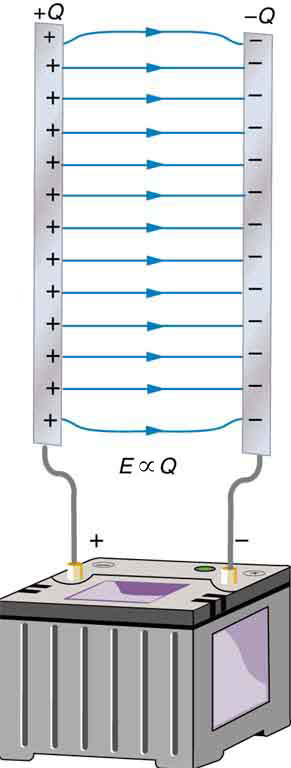E = V/d = F/q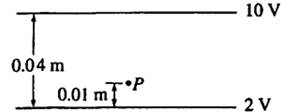Conducting plates are 0.04 m apart, as shown above.  Point P is located 0.01 m above the lower plate.

a. The electric potential at point P is

(A) 10 V (B) 8 V (C) 6 V (D) 4 V (E)  2 VE = V/d = 8 V/.04m

= 200 V/m

200 V/m = V/.01m

V = 2 V

(A) 10 V (B) 8 V (C) 6 V (D) 4 V (E)  2 Vb. The magnitude of the electric field at point P is

(A)  800 V/m (B)  600 V/m          (C)  400 V/m (D)  200 V/m          (E)  100 V/mE =V/d = 2 VCapacitance

Capacitor -

a) Oppositely charged plates connected to a voltage source

b) used to store charge

c) once capacitor is charged, voltage source can be removed

Q = CV

Q - charge

V - Voltage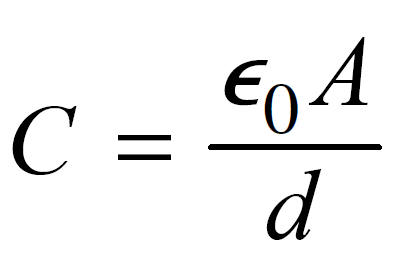A = Area of each plate

d - distance between plates

E0 = epsilon (ref)

= 8.84 x 10-12 C/VmMillikan